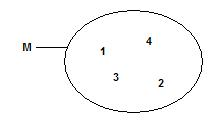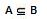# How is the set defined in mathematical terms?

## Quantities and elements

Sets and elements are part of the basics of mathematics. In this context, we also deal with the representation of sets, subsets, union sets, etc. What these terms are all about is covered in this article.Not only in real life, but also in mathematics, one tries to put things into categories. For this reason, one has the concept of in mathematics amounts introduced. For example, the numbers 1, 2, 3, 4 etc. belong to the set of natural numbers.

Show:

### Representation of quantities

Let's look at another example: The object x belongs to the set M. Mathematically speaking, this is written as follows: xεM. If an object does not belong to a set, we use the element symbol ε simply crossed out in the middle. In the following, four numbers are assigned to a set: M = {1, 2, 3, 4}. The numbers that belong to a set are written in curly brackets. A graphical solution also exists for this example and looks like this:

Table scrollable to the rightA few more examples should further clarify the principle of sets:

• The set of natural numbers: M = {0, 1, 2, 3, 4, 5, 6 ...}
• M1 = {x | x is a real number and solution of the equation x2 = 1} = {-1, 1}
• M2 = {x | x is a natural number with -2

More terms

A number of terms have been introduced around quantities, which we would now like to draw your attention to.

• Empty sets: The empty set contains no element, not even zero.
• Equality of sets: Two sets A and B are said to be the same if every element of A is also an element of B and every element of B is also an element of A.
• Power of sets: Two sets X and Y are equal if there is a clear mapping of the elements from X to the elements of Y, i.e. if exactly one element from Y can be assigned to each element from X.
• Finite sets are equal if they have the same number of elements
• Subset: The set A is a partial set of the set B, if every element of A is contained in B at the same time. B is then called the superset of A.
• Notation:• Example: A is the set of all sixth grade students. B is the set of all students in this school. So every element of A is also an element of B.
• Real subset: Is every element of A also contained in B and exists in B at least an element which is not contained in A, then A is a real subset of B.
• Non-element (disjoint) sets: Two sets X and Y are disjoint (foreign to element) if they have no element in common.
• Example: The set of natural numbers without zero and the set of negative numbers are not elementary.
Show:

### Set operations

As Set operation the connection of two sets is called. A new set is formed from the elements of the initial set. Here, too, several mathematical terms have been defined:

• Union set: If you combine the elements of two sets, you get the union set.
• Example: The set A = {1, 2, 3, 4} and the set B = {1, 5, 6, 7} are combined and called set C. This then looks like this: C = {1, 2, 3, 4, 5, 6, 7}.
• Intersection: An intersection includes all elements that are present in both sets.
• Example: The intersection C is formed for the sets A = {1, 2, 3, 4} and B = {2, 4, 6, 7}. This looks like this: C = {2, 4}
• Difference amount: The difference set (remainder) A \ B of two sets A and B is the set of all elements that belong to A but not to B.
• Example: We have the sets A = {1, 5, 7, 10} and B = {0, 1, 7, 15} and form the difference set A \ B = {5, 10}

Left:

### Who's Online

We have 1177 guests online